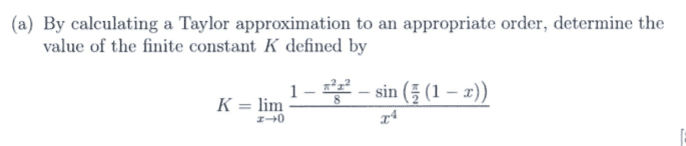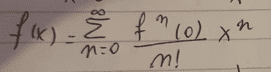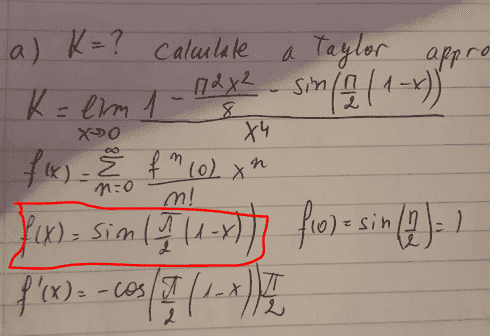# By calculating a Taylor approximation, determine K

• Jozefina Gramatikova

## Homework Statement## Homework Equations[/B]

## The Attempt at a SolutionCan somebody explain to me how did we find the function in red? Thanks

#### Attachments

I recommend you first expand this function using ## \sin(\theta-\phi)=\sin(\theta) \cos(\phi)-\sin(\phi) \cos(\theta) ##, and then work a Taylor series after putting in the values for ## \theta ## and ## \phi ##.

Assuming you did the above step, what do you get for the Taylor expansion of ## \cos(\frac{\pi}{2} x) ##?

I'm going to give you a couple more hints on this problem, because it is really quite a neat one: ## \\ ## Let ## f(x)=\cos(\frac{\pi}{2}x )##. This can be written as ## f(x)=\cos(bx) ## where ## b=\frac{\pi}{2} ##. Now, by the chain rule, ## f'(x)=-b \sin(bx) ##, and ## f''(x)=-b^2 \cos(bx) ##. The Taylor series ## f(x)=f(0)+f'(0)(x-0)+f''(0) \frac{(x-0)^2}{2!}+... ## ## \\ ## I gave you a couple of the terms here to get you started, but this problem actually will require even the 4th derivative. (Compute these first couple of terms and you will see why).